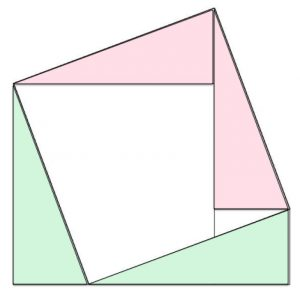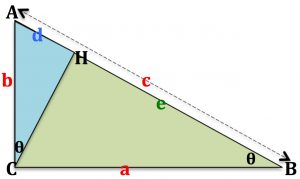There are hundreds of proofs of Pythagoras Theorem.

This diagram shows a proof.

Look carefully. Can you see three squares?

Assuming that they are squares, can you prove that four of the triangles are congruent?

Can you explain the proof?

The second diagram shows a well known proof using similar triangles.Angle ACB is a right angle and CH is perpendicular to AB.

Explain why the two angles labelled $$\theta$$ must be equal.

Explain why $$b^2 = dc \quad (1)$$.

Explain why $$a^2 = ec \quad (2)$$.

Combine equations (1) and (2) to give a proof of Pythagoras Theorem.